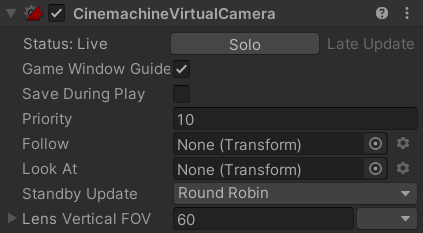# I can't change the FOV of my Camera in my script

I have a static camera in my scene and a player. When the player moves forward, I want the FOV of the static camera to increase.

Even if the float cameraFOV is changing (I saw it in the console), the real FOV of the camera isn’t changing.Can someone help me ? Here’s my code :

``````    public Transform targetObject;

public float rotationSpeed = 1.0f;
public float FOVSpeed;

private float previousXPosition;
private float previousYPosition;
private float previousZPosition;

private float cameraFOV;

void Start()
{
previousXPosition = targetObject.position.x;
previousYPosition = targetObject.position.y;
previousZPosition = targetObject.position.z;

cameraFOV = GetComponent<CinemachineVirtualCamera>().m_Lens.FieldOfView;
}

void Update()
{
float currentXPosition = targetObject.position.x;
float currentYPosition = targetObject.position.y;
float currentZPosition = targetObject.position.z;

if (currentXPosition < previousXPosition) //When player moves forward, FOV increases
{
float FOVAmount = (previousXPosition - currentXPosition) * FOVSpeed;
cameraFOV += FOVAmount;

}
if (currentXPosition > previousXPosition)//When player moves backward, FOV decreases
{
float FOVAmount = (currentXPosition - previousXPosition) * FOVSpeed;
cameraFOV -= FOVAmount;

}

if (currentYPosition > previousYPosition)//When player jumps, camera rotates down
{
float rotationAmount = (currentYPosition - previousYPosition) * rotationSpeed;
transform.Rotate(new Vector3(rotationAmount, 0, 0));
}
if (currentYPosition < previousYPosition)//When player lands, camera rotates up
{
float rotationAmount = (previousYPosition - currentYPosition) * rotationSpeed;
transform.Rotate(new Vector3(-rotationAmount, 0, 0));
}

if (currentZPosition > previousZPosition)//When player moves right, camera rotates left
{
float rotationAmount = (previousZPosition - currentZPosition) * rotationSpeed;
transform.Rotate(new Vector3(0, rotationAmount, 0));
}
if (currentZPosition < previousZPosition)//When player moves left, camera rotates right
{
float rotationAmount = (currentZPosition - previousZPosition) * rotationSpeed;
transform.Rotate(new Vector3(0, -rotationAmount, 0));
}

previousXPosition = currentXPosition;
previousYPosition = currentYPosition;
previousZPosition = currentZPosition;
}
``````